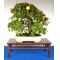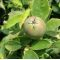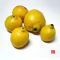##### The Japanese Bonsai specialist
Direct order Contact Help / Services Newsletter# Seeds of pseudo cydonia sinensis

Karinref. : 56,00

Available quantity : 16Order

###### Description

Sachet of 50 seeds of pseudo cydonia sinensis harvested freshly in Japan. The quince is appreciated of the professionels and Japanese amateurs for his/her/its rosy foliage in the spring becomes of a green quick in summer and red orange fire in fall, but also for the beauty of his/her/its peel and the burst of his/her/its yellow fruits. Instructions for use include in the sachet. The fast growth of this species allows you to form some forests, of the mini bonsai in a short time. It is indispensable to choose the style of tree since the end of the first year of culture.

#seeds 5.5 #sinensis 3.8 #cydonia 3.7 #pseudo 3.6 #sachet 2.6 #acer 2.4 #more 2.4 #professionels 2.3 #indispensable 2.3 #instructions 2.2

Formule
(( ROUND((CHAR_LENGTH(b.article_nom)-CHAR_LENGTH(REPLACE(b.article_nom, 'sinensis', '')))/LENGTH('sinensis')) + ROUND((CHAR_LENGTH(b.article_description)-CHAR_LENGTH(REPLACE(b.article_description, 'sinensis', '')))/LENGTH('sinensis')) ) * 3.8) + (( ROUND((CHAR_LENGTH(b.article_nom)-CHAR_LENGTH(REPLACE(b.article_nom, 'cydonia', '')))/LENGTH('cydonia')) + ROUND((CHAR_LENGTH(b.article_description)-CHAR_LENGTH(REPLACE(b.article_description, 'cydonia', '')))/LENGTH('cydonia')) ) * 3.7) + (( ROUND((CHAR_LENGTH(b.article_nom)-CHAR_LENGTH(REPLACE(b.article_nom, 'pseudo', '')))/LENGTH('pseudo')) + ROUND((CHAR_LENGTH(b.article_description)-CHAR_LENGTH(REPLACE(b.article_description, 'pseudo', '')))/LENGTH('pseudo')) ) * 3.6) + (( ROUND((CHAR_LENGTH(b.article_nom)-CHAR_LENGTH(REPLACE(b.article_nom, 'seeds', '')))/LENGTH('seeds')) + ROUND((CHAR_LENGTH(b.article_description)-CHAR_LENGTH(REPLACE(b.article_description, 'seeds', '')))/LENGTH('seeds')) ) * 3.5) + (( ROUND((CHAR_LENGTH(b.article_nom)-CHAR_LENGTH(REPLACE(b.article_nom, 'sachet', '')))/LENGTH('sachet')) + ROUND((CHAR_LENGTH(b.article_description)-CHAR_LENGTH(REPLACE(b.article_description, 'sachet', '')))/LENGTH('sachet')) ) * 2.6) + (( ROUND((CHAR_LENGTH(b.article_nom)-CHAR_LENGTH(REPLACE(b.article_nom, 'indispensable', '')))/LENGTH('indispensable')) + ROUND((CHAR_LENGTH(b.article_description)-CHAR_LENGTH(REPLACE(b.article_description, 'indispensable', '')))/LENGTH('indispensable')) ) * 2.3) + (( ROUND((CHAR_LENGTH(b.article_nom)-CHAR_LENGTH(REPLACE(b.article_nom, 'professionels', '')))/LENGTH('professionels')) + ROUND((CHAR_LENGTH(b.article_description)-CHAR_LENGTH(REPLACE(b.article_description, 'professionels', '')))/LENGTH('professionels')) ) * 2.3) + (( ROUND((CHAR_LENGTH(b.article_nom)-CHAR_LENGTH(REPLACE(b.article_nom, 'instructions', '')))/LENGTH('instructions')) + ROUND((CHAR_LENGTH(b.article_description)-CHAR_LENGTH(REPLACE(b.article_description, 'instructions', '')))/LENGTH('instructions')) ) * 2.2) + (( ROUND((CHAR_LENGTH(b.article_nom)-CHAR_LENGTH(REPLACE(b.article_nom, 'appreciated', '')))/LENGTH('appreciated')) + ROUND((CHAR_LENGTH(b.article_description)-CHAR_LENGTH(REPLACE(b.article_description, 'appreciated', '')))/LENGTH('appreciated')) ) * 2.1) + (( ROUND((CHAR_LENGTH(b.article_nom)-CHAR_LENGTH(REPLACE(b.article_nom, 'harvested', '')))/LENGTH('harvested')) + ROUND((CHAR_LENGTH(b.article_description)-CHAR_LENGTH(REPLACE(b.article_description, 'harvested', '')))/LENGTH('harvested')) ) * 1.9)

## Secure payment## Delivery

Our logistic partners :